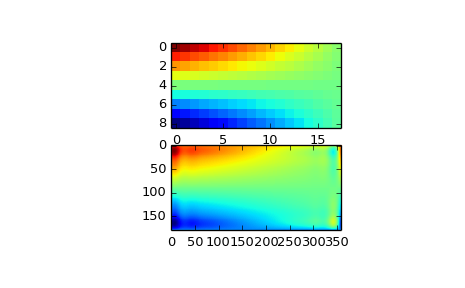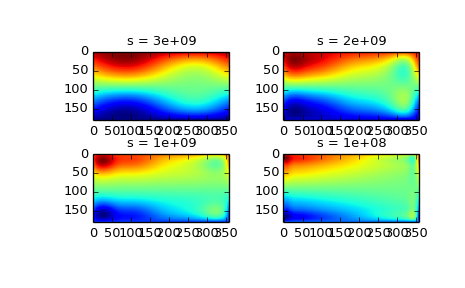# scipy.interpolate.RectSphereBivariateSpline¶

class scipy.interpolate.RectSphereBivariateSpline(u, v, r, s=0.0, pole_continuity=False, pole_values=None, pole_exact=False, pole_flat=False)[source]

Bivariate spline approximation over a rectangular mesh on a sphere.

Can be used for smoothing data.

New in version 0.11.0.

Parameters: u : array_like 1-D array of latitude coordinates in strictly ascending order. Coordinates must be given in radians and lie within the interval (0, pi). v : array_like 1-D array of longitude coordinates in strictly ascending order. Coordinates must be given in radians, and must lie within (0, 2pi). r : array_like 2-D array of data with shape (u.size, v.size). s : float, optional Positive smoothing factor defined for estimation condition (s=0 is for interpolation). pole_continuity : bool or (bool, bool), optional Order of continuity at the poles u=0 (pole_continuity) and u=pi (pole_continuity). The order of continuity at the pole will be 1 or 0 when this is True or False, respectively. Defaults to False. pole_values : float or (float, float), optional Data values at the poles u=0 and u=pi. Either the whole parameter or each individual element can be None. Defaults to None. pole_exact : bool or (bool, bool), optional Data value exactness at the poles u=0 and u=pi. If True, the value is considered to be the right function value, and it will be fitted exactly. If False, the value will be considered to be a data value just like the other data values. Defaults to False. pole_flat : bool or (bool, bool), optional For the poles at u=0 and u=pi, specify whether or not the approximation has vanishing derivatives. Defaults to False.

See also

RectBivariateSpline
bivariate spline approximation over a rectangular mesh

Notes

Currently, only the smoothing spline approximation (iopt = 0 and iopt = 1 in the FITPACK routine) is supported. The exact least-squares spline approximation is not implemented yet.

When actually performing the interpolation, the requested v values must lie within the same length 2pi interval that the original v values were chosen from.

For more information, see the FITPACK site about this function.

Examples

Suppose we have global data on a coarse grid

>>> lats = np.linspace(10, 170, 9) * np.pi / 180.
>>> lons = np.linspace(0, 350, 18) * np.pi / 180.
>>> data = np.dot(np.atleast_2d(90. - np.linspace(-80., 80., 18)).T,
...               np.atleast_2d(180. - np.abs(np.linspace(0., 350., 9)))).T


We want to interpolate it to a global one-degree grid

>>> new_lats = np.linspace(1, 180, 180) * np.pi / 180
>>> new_lons = np.linspace(1, 360, 360) * np.pi / 180
>>> new_lats, new_lons = np.meshgrid(new_lats, new_lons)


We need to set up the interpolator object

>>> from scipy.interpolate import RectSphereBivariateSpline
>>> lut = RectSphereBivariateSpline(lats, lons, data)


Finally we interpolate the data. The RectSphereBivariateSpline object only takes 1-D arrays as input, therefore we need to do some reshaping.

>>> data_interp = lut.ev(new_lats.ravel(),
...                      new_lons.ravel()).reshape((360, 180)).T


Looking at the original and the interpolated data, one can see that the interpolant reproduces the original data very well:

>>> import matplotlib.pyplot as plt
>>> fig = plt.figure()
>>> ax1 = fig.add_subplot(211)
>>> ax1.imshow(data, interpolation='nearest')
>>> ax2 = fig.add_subplot(212)
>>> ax2.imshow(data_interp, interpolation='nearest')
>>> plt.show()Chosing the optimal value of s can be a delicate task. Recommended values for s depend on the accuracy of the data values. If the user has an idea of the statistical errors on the data, she can also find a proper estimate for s. By assuming that, if she specifies the right s, the interpolator will use a spline f(u,v) which exactly reproduces the function underlying the data, she can evaluate sum((r(i,j)-s(u(i),v(j)))**2) to find a good estimate for this s. For example, if she knows that the statistical errors on her r(i,j)-values are not greater than 0.1, she may expect that a good s should have a value not larger than u.size * v.size * (0.1)**2.

If nothing is known about the statistical error in r(i,j), s must be determined by trial and error. The best is then to start with a very large value of s (to determine the least-squares polynomial and the corresponding upper bound fp0 for s) and then to progressively decrease the value of s (say by a factor 10 in the beginning, i.e. s = fp0 / 10, fp0 / 100, ... and more carefully as the approximation shows more detail) to obtain closer fits.

The interpolation results for different values of s give some insight into this process:

>>> fig2 = plt.figure()
>>> s = [3e9, 2e9, 1e9, 1e8]
>>> for ii in xrange(len(s)):
...     lut = RectSphereBivariateSpline(lats, lons, data, s=s[ii])
...     data_interp = lut.ev(new_lats.ravel(),
...                          new_lons.ravel()).reshape((360, 180)).T
...     ax = fig2.add_subplot(2, 2, ii+1)
...     ax.imshow(data_interp, interpolation='nearest')
...     ax.set_title("s = %g" % s[ii])
>>> plt.show()Methods

 __call__(theta, phi[, dtheta, dphi, grid]) Evaluate the spline or its derivatives at given positions. ev(theta, phi[, dtheta, dphi]) Evaluate the spline at points Returns the interpolated value at (theta[i], phi[i]), i=0,...,len(theta)-1. get_coeffs() Return spline coefficients. get_knots() Return a tuple (tx,ty) where tx,ty contain knots positions of the spline with respect to x-, y-variable, respectively. get_residual() Return weighted sum of squared residuals of the spline

#### Previous topic

scipy.interpolate.RectBivariateSpline.integral

#### Next topic

scipy.interpolate.RectSphereBivariateSpline.__call__